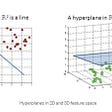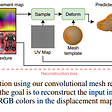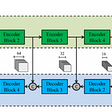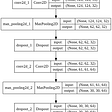# Neural Networks 101 — Part 2

## Getting hands dirty with code

Hope you guys read the previous part of this blog, where we discussed the following topics,

• When do we call a ‘program’ a machine learning model?
• How to convert a traditional program into a machine learning model
• 7 steps

If you didn't read the previous part, make sure to check it out here Neural Networks 101 — Part 1 because that acts as a base for what you gonna see in this part. We’ve played enough with the theory, now it's time to get our hands dirty with code. In this part, we will write PyTorch and fastai code to represent the 7 steps with actual data called MNIST.

## What can you expect

• Learn tidbits of Fastai and Pytorch
• Understanding how the code works
• Learn how to calculate gradients
• Building a complete model with MNIST data
• A complete executable notebook.

Note: The notebook version of this blog is available here Neural Networks 101 Google Colab, feel free to run the cells and visualize the results.

Enough of talking let's jump right in, before getting started we gotta make sure about the data even though we gonna deal with this later let's load them.

`# Loading the mnist data and untar itdata_path = untar_data(URLs.MNIST)`

In the above code cell, we have just downloaded the MNIST data, and the function `untar_data` takes care of the download and returns us a path of where the files are stored.

We will leave it here, for now, let us see how to calculate gradients with Pytorch.

As we know what are gradients and why they are important let's see how to code them using Pytorch. First will take a look at the whole code, then will gradually break down every line and see what they do.

• `xt = tensor(8.).requires_grad_()` : creates a tensor at first, and by setting requires_grad_() True to any tensor in Pytorch will automatically track and calculate gradients for that tensor.
• And we know the next step we will apply some computations with our tensor, then, at last, activate the backpropagation which helps us in getting the gradients.
• `yt.backward()` : this will calculate the gradients by activating the backpropagation.
• `xt.grad` : will give us the gradients calculated on this variable during the computation.

But you might be thinking why do we use require_grads_() and what's the underlying mechanism behind this calculation. In my recent blog, I explained auto differentiation and its underlying mechanism that powers up this whole thing.

## Glimpse on Auto Differentiation

Well, the first time when we are computing our loss function with our parameters it will return the partial derivatives and we call this process forward pass.

The forward pass is responsible for computing the loss function with our parameters. But we know that a neural network has to optimize its parameters to achieve the best results and that is getting a minimized loss error.

But how do we find the values that will help the neural network to find the best parameters to minimize the loss?

We have to get the gradients by activating the backpropagation (or) back pass. At first, we performed a forward pass and got our partial derivatives, and by activating the backpropagation that uses the chain rule to compute the gradients for us.

But what do all of these things have to do with auto differentiation?

Auto differentiation helps us to keep track of these computations and during the backpropagation, it just has to use these parameters to compute the gradients. And we know just with the help of partial derivatives we were able to compute the gradients of the trainable variables (weights and biases) and still able to keep a record of thousands of derivatives and gradients.

Note: The gradients will tell us only the slope of our function, they don’t really say how far we should adjust the parameters.

So how to tell our parameters the way they should move to minimize the loss?

We will use something called the learning rate.

The gradients tell us the directions but not the magnitude of the direction (i.e the step we have to take). This is where our learning rate helps, it tells us how large each step should be (or) in other words it gives us the scale of how much we should trust the gradients and step in the direction of that gradient.

So we will multiply the gradient by a small number (learning rate) to step the weights.

# And End-to-End SGD Example

We are the fun part now. Let's code the seven-step we discussed in our previous part of the blog. Before jumping into the code let's re-visit the seven steps,

• Step 1: Find a way to initialize random weights.
• Step 2: And for each image, use these weights to predict whether it appears to be a `3` or a `7`.
• Step 3: Based on the above predictions calculate how good the model is. This is where we introduce the term called loss function.
• Step 4: Calculate the gradient, which plays a crucial role in weight assignment. It will tell us how to change the weights so that our loss would change.
• Step 5: Step the weights, that is change the weights based on the gradients calculated.
• Step 6: Go back to Step 2 and repeat the process.
• Step 7: Iterate until we decide to stop the training process.

Now comes the code!

As we know the first 4 steps are very similar and straightforward, so I won't talk about that.

• `params.data -= lr * params.grad.data` : here we multiply our learning to our gradients and update the values. A special method tells PyTorch we want to calculate gradients w.r.t to the variable at the value. (xt → variable , 3 → value)
• `params.grad = None` : making the gradients zero so it won't add up with the previous existing gradients.

Let's create some dummy data and use our above function for the training.

It's fine if some of the code doesn't make sense because the whole point of this blog is focused on the gradients and the workflow that takes place during the process. Like I said before the notebook contains code packed in and people can execute it sequentially and visualize the results.

# Wrapping up with Fastai

Let's give a final touch to this blog by wrapping up with actual data and train a model that recognizes digits. Rather than using the mid-level components of Fastai in this blog, we will stick strictly with the low-level API and create a model with that.

Also, it's fine if the code doesn't make sense, I just wanna show people how you can use Fastai + Pytorch to build models.

Let's break down the above code,

## `Datasets`

The Datasets expects,

• the items we want to use
• the transforms (how the inputs and outputs should be constructed and spits out)
• the type of split (train and test)

Decoding the `dsets` :

• `PILImageBW` -> creates a PIL image (accepts a file path)
• `.create` -> takes care of the preprocessing before going into the model. This is applicable for both X and y, more like a custom implementation for the various inputs.
• `splits` itself doesn't do the splitting, we've just created an instance of the object, where passing the items later will give us the train and test sets.

## Transforms

We got our filenames converted into images, but for a machine learning model, we have to convert our images into tensors (numerical representation) and make it easy for our model to learn patterns on it.

We need to give ourselves some transforms on the data! These will need to:

• Ensure our images are all the same size
• Make sure our output is the tensor our models are wanting
• Give some image augmentation
`# Creating transforms for our data by hand (left to right)tfms = [ToTensor() , CropPad(size = 34 , pad_mode = PadMode.Zeros) , RandomCrop(size = 28)]`

We need one more thing, at last, that is the transforms applied during the GPU instance or in other words, transforms applied for every batch.

The important reason for having mini-batches is they could run on GPU, so the computations take place even faster. Also batching prevents bias during training and helps the training converge faster.

We have to load our Datasets into a `DataLoaders` so it will help us to batch our data and sends a batch of our whole data during the training time.

`# Creating the batch transformsgpu_tfms = [IntToFloatTensor() , Normalize()]# Building our dataloadersdls = dsets.dataloaders(bs = 128 , after_item= tfms , after_batch= gpu_tfms)`

Let's visualize our images.

Look at that, how beautiful it is? From file paths to actual images we’ve come a long way!

But we’ve reached our goal for this blog and the next step is creating and fitting the model. This wrapping up section is more like a shoutout to the amazing Fastai people, without them this blog wouldn't be possible in the first place.

After building and training our model for 3 epochs or 3 iterations we will have around 98% accuracy, which means our model is doing a pretty good job of recognizing the digits.

It's advisable to look into the notebook version of this blog to get your hands dirty with the code. Links for the resource are given below. Until then,

Happy Learning!

Artificialis: Discord community server, full of AI enthusiasts and professionals.
Also our
Newsletter, weekly updates on my work, news in the world of AI, tutorials, and more!

--

--

--

## More from Artificialis

A home for Data Science and Machine Learning. Share ideas and concepts with us.

## 50 of the most profound Kaggle Discussions (tips, tricks, resources) by the top Kaggle Grandmasters## DCGAN (Deep Convolutional GAN)## NLP: Sentiment Analysis or Emotion Mining on Amazon Product Reviews - Part-1## Recognizing Handwritten Digits with scikit-learn## Review of Two NIPS 2020 Papers on 3D Reconstructions from 2D Images.## 文章閱讀 Learning and Adapting Robust Features for Satellite Image Segmentation on Heterogeneous Data…## 20 Beginner-Friendly Image Datasets to Hone Your Computer Vision Skills## Do Neural Networks Dream of Electric Sheep?## Ashik Shaffi

Machine Learning Practitioner

## Python Implementation of Gradient Descent and Its Variants (Part 2)## Transfer learning and active learning to find images of a small class in an unlabeled dataset.## RajeshDai Detector: Creating a Simple Binary Image Classifier with Neural Networks## Activation Functions